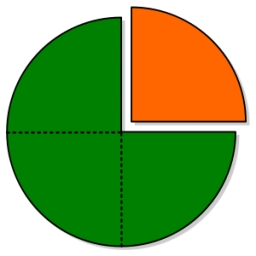# One quarter

Which of the following has a sum of 3/4?

A. 1/2+1/4
B. 1/2+1/3
C. 1/4+1/8
D. 1/9+1/12​

Result

x = (Correct answer is: A)### Step-by-step explanation:Did you find an error or inaccuracy? Feel free to write us. Thank you!

Tips for related online calculators
Need help calculating sum, simplifying, or multiplying fractions? Try our fraction calculator.